# 史上最强副驾驶——开车打瞌睡？Python叫醒你### 驾驶员疲劳检测系统

• 第1步-从相机中获取图像作为输入。
• 第2步-检测图像中的脸部并创建一个感兴趣区（ROI）。
• 第3步-从ROI中检测人眼并将其输入分类器。
• 第4步-分类器将按睁开或闭合对人眼进行分类。
• 第5步-计算分数判断驾驶员是否处于疲劳状态。

### 先决条件

• OpenCV – 使用pip安装opencv-python（面部和眼部检测）。
• TensorFlow – 使用pip安装tensorflow（keras使用TensorFlow作为后端）。
• Keras – 使用pip安装keras（建立分类模型）。
• Pygame – 使用pip安装pygame（播放警告提示音）。

### 数据集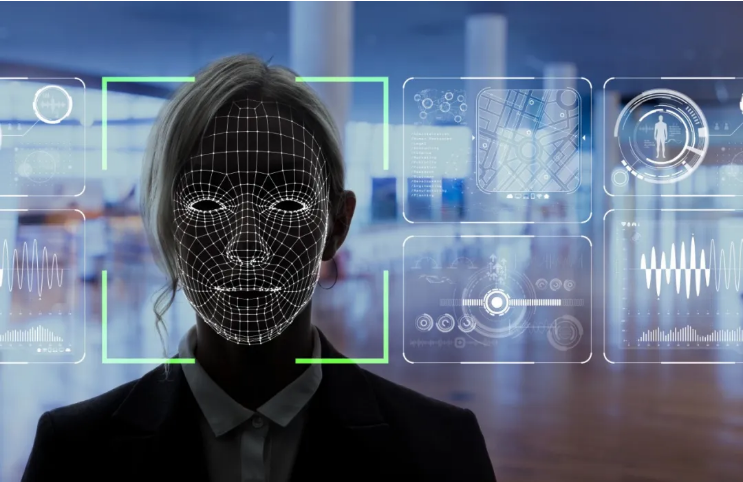### 模型结构

CNN基本上由一个输入层、一个输出层和一个可以包含多个层的隐藏层组成。通过过滤器在这些层上执行卷积运算，该滤波器在层和过滤器上执行2D矩阵乘法。

CNN模型结构包括以下几层：

• 卷积层；32个节点，内核大小为3
• 卷积层；32个节点，内核大小为3
• 卷积层；64个节点，内核大小为3
• 完全连接层；128个节点

### Python项目进行驾驶员疲劳检测的步骤

zip的目录为：

2.模型文件夹包含我们的模型文件“cnnCat2.h5”，该文件是在卷积神经网络上进行训练的。

3.我们有一段音频“alarm.wav”，用于在驾驶员昏昏欲睡时播放。

4.“Model.py”文件包含一个程序，通过该程序对数据集进行训练来构建分类模型。您可以从此文件中了解卷积神经网络的执行情况。

5.“Drowsinessdetection.py”是本项目的主要文件。开始检测时，我们必须要运行此文件。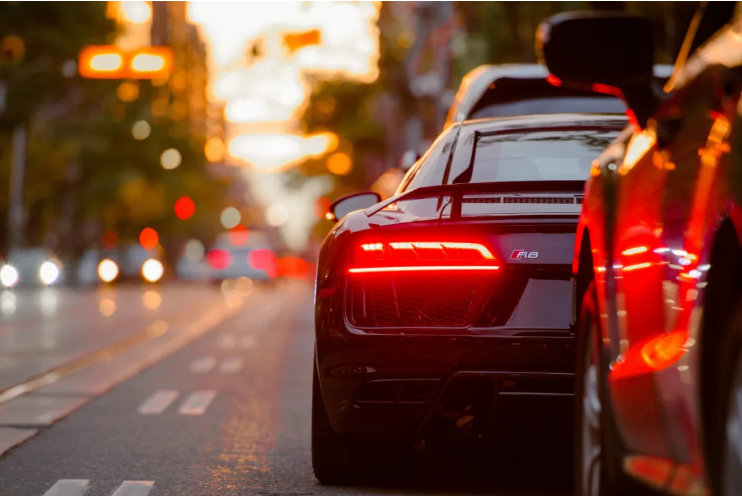``````for (x,y,w,h)in faces:cv2.rectangle(frame,(x,y), (x+w, y+h), (100,100,100), 1 )
``````

``````l_eye =frame[ y : y+h, x : x+w ]
``````

l_eye仅包含左眼的图像数据。这将被输入到CNN分类器中，该分类器将预测眼睛是处于睁开还是闭合状态。同样，我们将右眼的数据提取到r_eye中。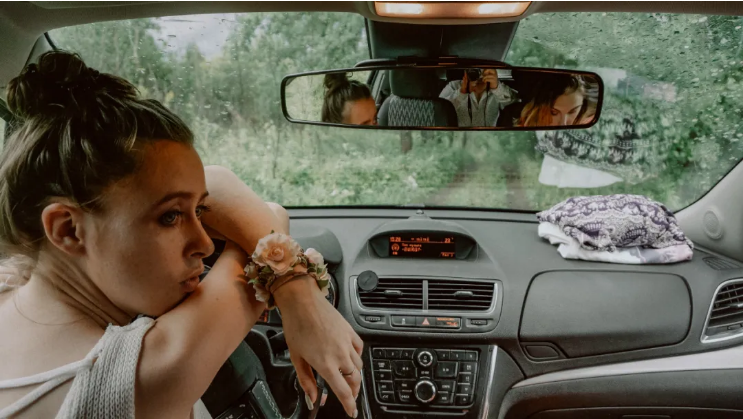``````cv2.putText(frame,“Open”, (10, height-20), font, 1, (255,255,255), 1, cv2.LINE_AA )
``````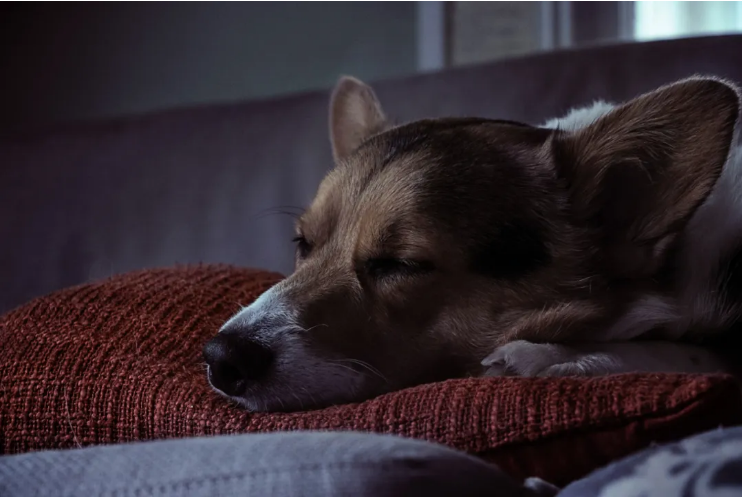``````import cv2
import os
fromkeras.models
import numpy asnp
from pygameimport mixer
import time
mixer.init()
sound =mixer.Sound( alarm.wav )
lbl=[ Close , Open ]
path =os.getcwd()
cap = cv2.VideoCapture(0)
font =cv2.FONT_HERSHEY_COMPLEX_SMALL
count=0
score=0
thicc=2
rpred=
lpred=
while(True):
height,width = frame.shape[:2]
gray = cv2.cvtColor(frame,cv2.COLOR_BGR2GRAY)
faces =face.detectMultiScale(gray,minNeighbors=5,scaleFactor=1.1,minSize=(25,25))
left_eye = leye.detectMultiScale(gray)
right_eye = reye.detectMultiScale(gray)
cv2.rectangle(frame, (0,height-50) ,(200,height) , (0,0,0) , thickness=cv2.FILLED )
for (x,y,w,h) in faces:
cv2.rectangle(frame,(x,y) , (x+w,y+h) , (100,100,100) , 1 )
for (x,y,w,h) in right_eye:
r_eye=frame[y:y+h,x:x+w]
count=count+1
r_eye =cv2.cvtColor(r_eye,cv2.COLOR_BGR2GRAY)
r_eye = cv2.resize(r_eye,(24,24))
r_eye= r_eye/255
r_eye= r_eye.reshape(24,24,-1)
r_eye = np.expand_dims(r_eye,axis=0)
rpred = model.predict_classes(r_eye)
if(rpred==1):
lbl= Open
if(rpred==0):
lbl= Closed
break
for (x,y,w,h) in left_eye:
l_eye=frame[y:y+h,x:x+w]
count=count+1
l_eye = cv2.cvtColor(l_eye,cv2.COLOR_BGR2GRAY)
l_eye = cv2.resize(l_eye,(24,24))
l_eye= l_eye/255
l_eye=l_eye.reshape(24,24,-1)
l_eye = np.expand_dims(l_eye,axis=0)
lpred = model.predict_classes(l_eye)
if(lpred==1):
lbl= Open
if(lpred==0):
lbl= Closed
break
if(rpred==0 and lpred==0):
score=score+1
cv2.putText(frame,"Closed",(10,height-20), font,1,(255,255,255),1,cv2.LINE_AA)
# if(rpred==1 or lpred==1):
else:
score=score-1
cv2.putText(frame,"Open",(10,height-20), font,1,(255,255,255),1,cv2.LINE_AA)
if(score<0):
score=0
cv2.putText(frame, Score: +str(score),(100,height-20), font,1,(255,255,255),1,cv2.LINE_AA)
if(score>15):
#person is feeling sleepy so we beepthe alarm
cv2.imwrite(os.path.join(path, image.jpg ),frame)
try:
sound.play()
except: # isplaying = False
pass
if(thicc<16):
thicc= thicc+2
else:
thicc=thicc-2
if(thicc<2):
thicc=2
cv2.rectangle(frame,(0,0),(width,height),(0,0,255),thicc)
cv2.imshow( frame ,frame)
if cv2.waitKey(1) & 0xFF == ord( q ):
break
cap.release()
cv2.destroyAllWindows()
``````

### Python项目示例

``````python“drowsiness detection.py”
``````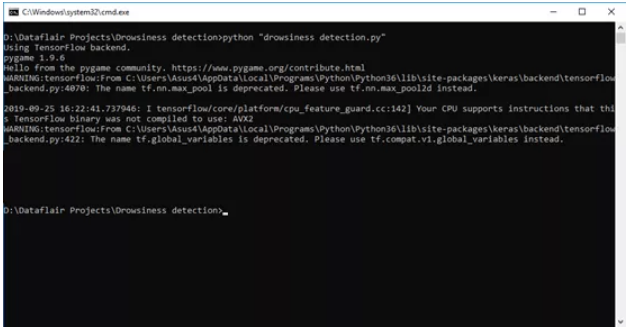1.闭眼检测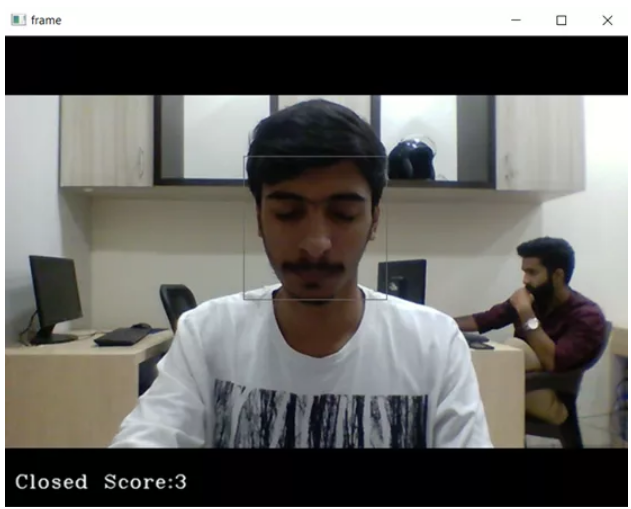2. 睁眼检测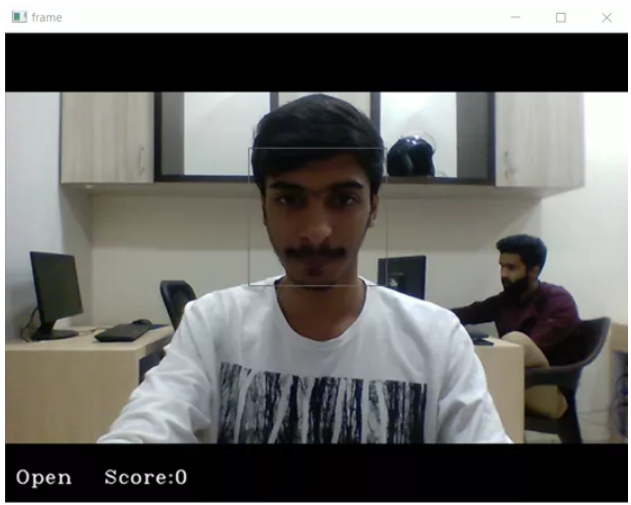3. 疲劳警告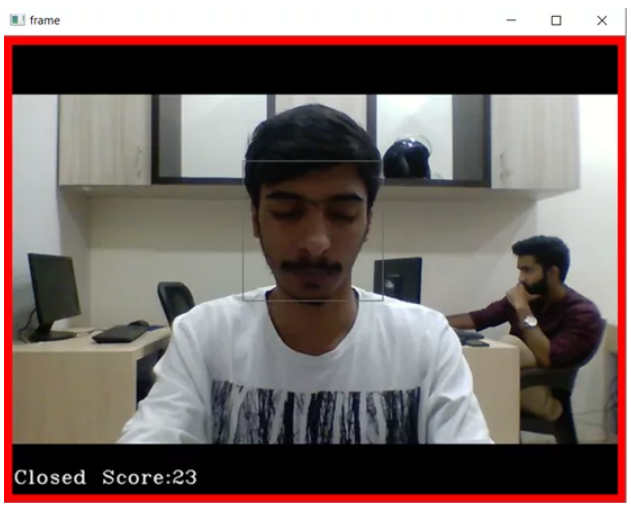【云栖号在线课堂】每天都有产品技术专家分享！

【云栖号在线课堂 社群】https://c.tb.cn/F3.Z8gvnK

### 最后编辑：2020/4/24编辑：AliParter